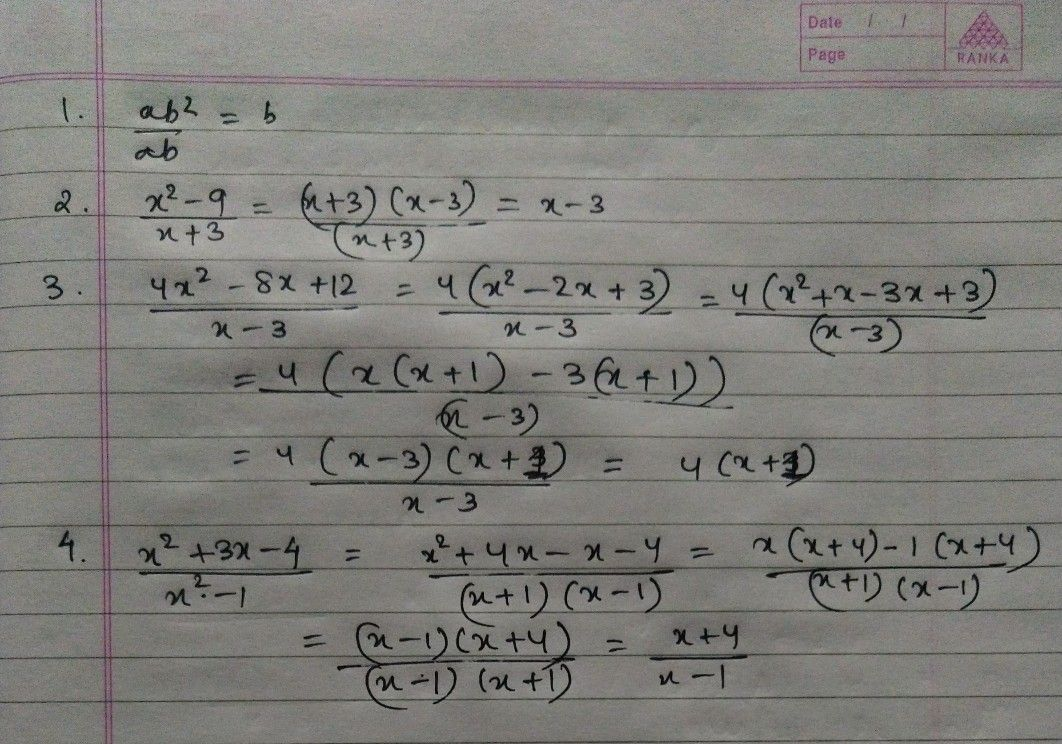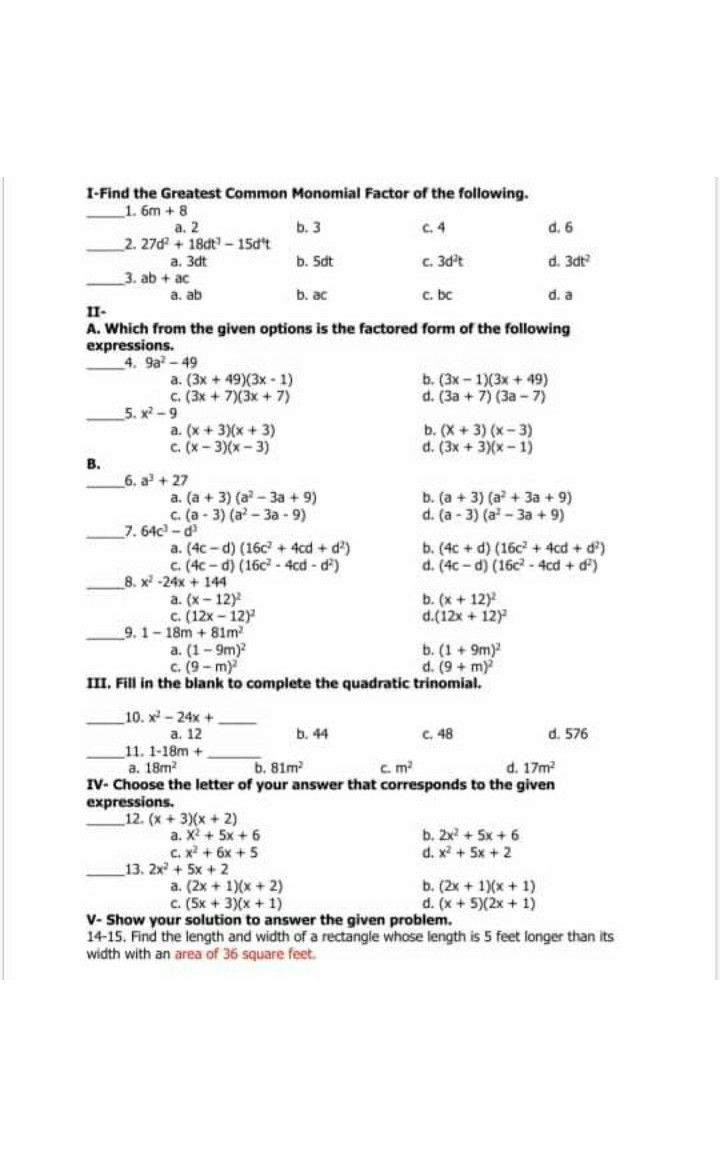Symbol
ProblemLearning Task $2$ Simplify the following rational expressions. Do $1$ it in your notebook $1$ $\dfrac {ab^{2}} {ab}$ $2$ $\dfrac {x^{2}-9} {x+3}$ $3$ $\dfrac {4x^{2}-8x+12} {x-3}$ $4$ $\dfrac {x^{2}+3x-4} {x^{3}-1}$
7th-9th grade
Algebra
Search count: 106
SolutionQanda teacher - AishwaryaStudent
how to get gcfQanda teacher - Aishwarya
those are the basic formulas
to solve quadratic equationsStudent